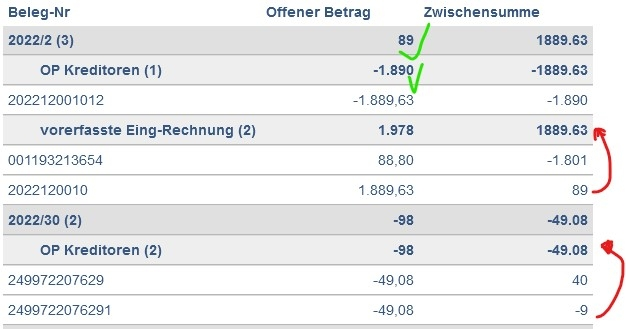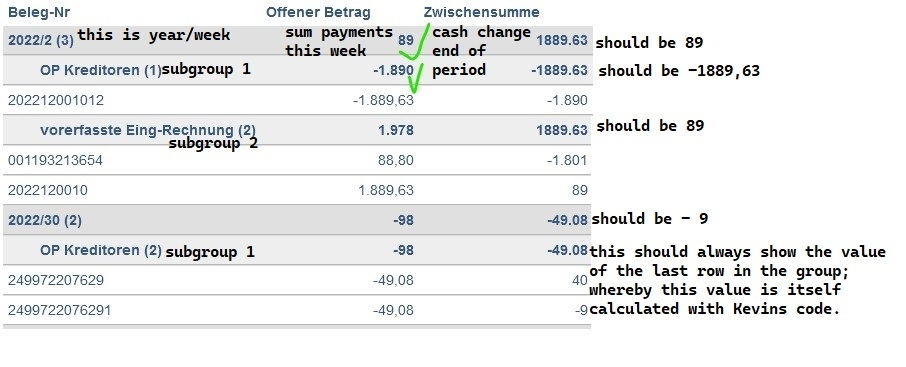# sort data before processing because of running total

Hi,

in my datatable i am having a runnung total like

``````            { data: "BUC_OPBETRAG", title: "runningsum",
render: function (data, type, row, meta ) {
if (meta.row == 0) {
zwisu = Number(data);
return (Number(data));
} else {
zwisu = zwisu + Number(data);
return (zwisu);
}
}
},
``````

which works well .But the data is not displayed in the order it is processed, so the running sum makes no sense at all.

I would have to order data - I believe befor it is processed - by the fields "calenderweek" and "source" - how can I achieve this?

Thanks
Max

• You'd need to do it before it is fed into DataTables. Are you Ajax loading the data or something else? If you could link to a test case showing how you are operating DataTables I'd be able to give a direct answer.

An alternative might be something like this example., but only if you don't care about the actual index assigned to a row, since that is applied regardless of order.

Allan

• Hi Allan,

I can not post a test case because we are in a safe environment....very sorry for that.

yes I get the data via ajax.

``````Editor::inst( \$db, 'Za_query', ['Ursprung', 'BUC_BUCHUNGID'])
->fields(
Field::inst( 'Ursprung' ),
Field::inst( 'BUC_BELEGID' ),
Field::inst( 'BUC_BUCHUNGID' ),
Field::inst( 'BUC_FIRMENNR' ),
Field::inst( 'ZahlungsPartner' ),
Field::inst( 'BUC_KONTONR' ),
Field::inst( 'Nettofaellig' ),
Field::inst( 'Skontofaellig' ),
Field::inst( 'BUC_ZES_ZESSKZ' ),
Field::inst( 'PEK_KUN_ZESSKZ' ),
Field::inst( 'Mahnsp_Beleg' ),
Field::inst( 'Mahnsp_Konto' ),
Field::inst( 'Faellig_seit_Tagen' ),
Field::inst( 'Mahnstufe' ),
Field::inst( 'KW_net' ),
Field::inst( 'KW_sko' )
)
->where( 'BUC_FIRMENNR', \$selectedFirma )

->debug(true)
->process( \$_POST )
->json();
``````

I also added a "running number" like this:

``````            { data: "BUC_OPBETRAG", title: "runningnumber",
render: function (data, type, row, meta ) {
if (meta.row == 0) {
zwinu = 1;
return (1);
} else {
zwinu = zwinu + 1;
return (zwinu);
}
}
},
``````

also this starts with 36, all numbers are mixed through.

So how can I achieve "You'd need to do it before it is fed into DataTables."? I tried to order them on the sql server, but that also does not work....

Thanks
Max

• The test case doesn't have to have your actual data since the problem is specific to your data. The test case just needs to represent what you are trying to do. I adapted Allan's example to show how to sum a column based on the order applied.
https://live.datatables.net/yidodabi/1/edit

If you want this to run only once then move the code inside the event handler to `initComplete`.

If you still need help then please build a simple test case that has an example of your data. If its easier create a Javascript sourced test case like this example. Also specify the order you want the summation to happen.
https://datatables.net/manual/tech-notes/10#How-to-provide-a-test-case

Kevin

• edited June 13

Are you client-side or server-side processing with this table (`serverSide`)?

I've been re-reading the question, and I'm not yet sure I 100% understand the goal. Do you want to always force the table to sort by `calenderweek` and `source` and then show an index column based on that order? Is the end user able to do apply custom ordering?

Or do you want the index to be in `calenderweek / source` order, and then the end user can order in any order they want (which would cause the index to be out of order, unless it was clicked on)?

Allan

• Hi Kevin, Hi Allan,

no, the user does not need custom ordering, so far Kevins solutions works perfect.
But (I seem do have a tendency to complicated tables) this table also has rowGroups. In the rowgroups I show the total of these rows (works), but now I would also need the last running total ("Zwischensumme") of this group! Aim is to see how much money will be left after each week.my rowgroup is defined:

``````       rowGroup: {
dataSrc: ["KW_net", "Ursprung" ],
startRender: function(rows, group, level) {
var api = \$('#table_orders').DataTable();
var all;

if (level === 0) {
top = group;
all = group;
} else if (level === 1) {
parent = top + group;
all = parent;
// if parent collapsed, nothing to do
if (collapsedGroups[top]) {return;}
} else {
// if parent collapsed, nothing to do
if (collapsedGroups[parent]) {return;}
all = top + parent + group;
}
var collapsed = collapsedGroups[all];

rows.nodes().each(function(r) {
r.style.display = collapsed ? 'none' : '';
});

var intVal = function ( i ) {
return typeof i === 'string' ?
i.replace(/[\\$,]/g, '') * 1 :
typeof i === 'number' ?
i : 0;
};
var numFormat = \$.fn.dataTable.render.number( '.', ',', 0, '' ).display;

sumoff = rows.data().pluck("BUC_OPBETRAG").toArray().reduce( function (a, b) {
return  intVal(a) + intVal(b);
}, 0 );
sumb = rows.data().pluck("BUC_OPBETRAG").toArray().reduce( function (a, b) {
return intVal(b);
}, 0 );
sumoff = numFormat(sumoff);

if(level==1){
\$platzhalter = "........";
\$bg_col = ";background-color: #eeeeee";
} else {
\$platzhalter = "";
\$bg_col = "";
}
return \$('<tr/>')
.append('<td colspan=1 style="' + \$bg_col + '"><p style="color: #eeeeee; display: inline-block; margin: 0px">'+ \$platzhalter + '</p>' + group + ' (' + rows.count() + ')</td><td colspan=1 style="text-align: right' + \$bg_col + '">' + sumoff +'</td><td colspan=1 style="text-align: right' + \$bg_col + '">' + sumb +'</td>')
//.append('<td colspan=99 style="' + \$bg_col + '"><div style="display: inline-block; margin: 0px; width: 70%"><p style="color: #eeeeee; display: inline-block; margin: 0px;">'+ \$platzhalter+'</p><p style="\$bg_col; display: inline-block; margin: 0px;">' + group+ '</p></div><p style="text-align: right; margin: 0px ;display: inline-block; width: 30%">' + sumoff + '|' + sumb + '</p></td>')
.attr('data-name', all)
.toggleClass('collapsed', level === 0 ? false : collapsed);
}
},
``````
• I'm not quite getting it - sorry. Could you modify the image to show what would be the expected values?

I'm not certain that what you are looking for will be possible, the grouping levels don't have a concept of higher levels, which I think you would need, but I'm not certain of.

Allan

• here you go.The idea is to have a cash-flow-planning. In the column "Offener Betrag" I see what is paid per week, in "Zwischensumme" I see the sum of all payments up to that week. I have two ideas how to get that value: either to take the value out of the last row of each group (if groups are inserted after all rows have been calculated), or (if groups are inserted while normal rows are also produced) taking the value out of Kevins calculation?

Thanks a lot for your effort!

• I'm not totally clear what you are after either. However if you are wanting to get the values from the parent group then I would look at creating a new variable, similar to the `collapsedGroups` variable that keeps track of the summed values for the group level. Then you can access them using `top` or `parent` variables as appropriate.

Maybe you can build a simple test case that shows a sample of what you have. Then we can collaborate on a solution.
https://datatables.net/manual/tech-notes/10#How-to-provide-a-test-case

Kevin

• I think I get it now based on your mocked up screenshot, but I'm afraid that is not something that DataTables will do. You could do it in `draw` and spin over the rows in the table, performing the calculations required, updating the cells, but it would not allow for search or ordering of that column.

Allan

no, the user does not need custom ordering

You'd need to do it before it is fed into DataTables.

yes I get the data via ajax.

Are you using Editor? In case you are you can manipulate the data quite easily server side and also implement complex sorting etc.

All you need to do is to manipulate "data" on "postGet".

In this example I add a total server side:

``````->on( 'postGet', function ( \$e, &\$data, \$id ) use ( \$lang ) {
if ( count(\$data) <= 0 ) {
return;
}
\$sum = array_sum(array_column(\$data, "unformattedAmount"));
\$keys = array_keys(\$data);
foreach ( \$keys as \$key ) {
unset(\$data[\$key]["unformattedAmount"]);
}
if ( \$sum != 0 ) {
\$data[] = [ "DT_RowId" => "row_0", //in reality there is no row 0 because it is derived from the SQL id
"sub_order_schedule" =>
[ "sub_order_id"   => \$_POST['sub_order_id'],
"payment_date"   => \$lang === "de" ? "Summe" : "Total",
"payment_amount" => getFormatterAmount(\$sum),
"voucher_number" => "",
}
})
``````

Here is another one with more complex sorting, elimination of duplicates and filtering. (Editor cannot do SELECT DISTINCT and ORDER BY - so I implement this using PHP).

``````->on( 'postGet', function ( \$e, &\$data, \$id ) use ( \$db ) {
\$data = array_unique(\$data, SORT_REGULAR);
\$stmt = ('SELECT DISTINCT govdept_id
FROM govdept_has_user
WHERE user_id = :user_id
\$result = \$db ->raw()
->bind(':user_id',\$_SESSION['id'])
->exec(\$stmt);
\$row = \$result->fetchAll(PDO::FETCH_ASSOC);
\$govdeptIdArray = [];
foreach (\$row as \$val) {
\$govdeptIdArray[] = \$val["govdept_id"];
}

foreach (\$data AS \$key => \$val) {
if ( ! in_array(\$val['dept_id'], \$govdeptIdArray) ) {
unset(\$data[\$key]);
}
}

\$data = array_values(\$data);
array_multisort( array_column(\$data, "affected_user"), SORT_ASC,
array_column(\$data, "dept_id"), SORT_ASC,
array_column(\$data, "update_time"), SORT_ASC,
\$data );
})
``````

Just to give you an idea how flexible this can be.

• Thanks for all the great inut. In the end I found a completely different solution. I set up a function I call in init-complete and after every table.draw. Works perfect.

`````` function tabelleaendern() {

var rows = document.querySelectorAll('.dtrg-group.dtrg-start.dtrg-level-0');
var rowsDet = document.querySelectorAll('#table_orders tr');

for (let p = 0; p < rows.length; p++) {
let row = rows[p];

var gruppe = row.cells.textContent;

///Detailszahl suchen
for (let x = 0; x < rowsDet.length; x++) {
let rowDet = rowsDet[x];

if (rowDet.cells.textContent == gruppe) {
var column0Value = rowDet.cells.textContent;
}
}
////ENDE Detailszahl

row.cells.textContent = (column0Value);
}
}
``````
• Nice one! Thanks for sharing your solution with us.

Allan